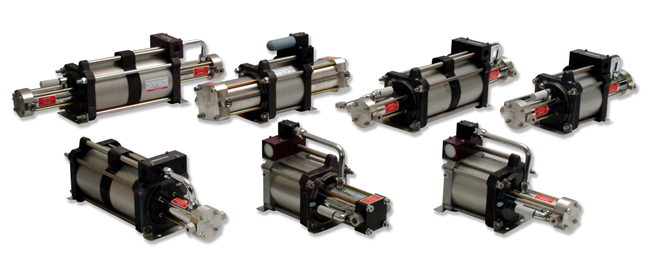# Guaranteed Savings with Maximator Air Amplifiers

November 3, 2014
###### Oxygen Gas Boosters: How Do They Work?
August 2, 2017

A variety of industries rely on compressed air operations to complete many applications. Manufacturing facilities utilize compressed air systems for processes such as increasing shop air supply pressure, improving pneumatic tool efficiency, and boosting air supply pressure for component removal processes.

Why Compressed Air Supply Pressure Operations are Expensive?

Supplying significant amounts of compressed air pressure requires a huge amount of energy. Generally, calculating average costs of compressed air systems is performed using the following formula:
\$\$ = [(HP) x (0.746 x (hours) x (\$/kW-hr) x (% working time) x (% full load)] / motor efficiency.

This formula can be better understood with an example. Let us suppose you have a compressed air system with the following specifications:

• Horsepower rating: 200 HP
• Working time: 6,800 hours
• Full Load (FL) percentage: 85%
• FL efficiency: .25
• Cost per kW-hr: 5¢

Therefore, using the above mentioned formula, we can calculate the total cost as:

• Full Load: \$\$ = [(200 HP) x (0.746 x (6,800) x (\$0.05) x (0.85%) x (1 FL)] / .95 = \$45,388.
• No Load: \$\$ = [(200 HP) x (0.746 x (6,800) x (\$0.05) x (0.15%) x (0.25 FL)] / .90 = \$2,113.

The total amount of energy consumed is the sum of full load + no load. Therefore,
\$45,388 + \$2,113 = \$47,502.

This makes for an expensive investment. Adding to this, these systems also suffer from problems such as air leaks, and inefficient system usage. Utilizing multiple systems running at higher outlet pressures than required can also lead to excess expenditure.Air Amplifiers – A Cost-Effective Solution

An air amplifier can provide a complete economical solution to all these problems. Maximator® air amplifiers have proven to be a reliable partner for the supply of air pressure in many industrial applications. High Pressure Technologies is one of the leading providers of Maximator® air amplifiers.

How do Maximator® Air Amplifiers provide Cost-Efficiency to Operations?

There are a number of reasons why Maximator® air amplifiers are most suitable for making compressed air supply pressure operations more economical. For air pressure reduction of 2 psi, the compressed air system HP is reduced by 1%. If we take the previous example, you will be able to see the benefits:

• If 200 HP is needed to maintain shop pressure of 120 psi, an air amplifier can reduce the shop air supply pressure down to 80 psi. That results in 20% less compressor HP consumption.
• Using the previously mentioned formula, the calculations show:
• Full Load: \$\$ = [(160 HP) x (0.746 x (6,800) x (\$0.05) x (0.85%) x (1% FL)] / .95 = \$36,310.
• No Load: \$\$ = [(160 HP) x (0.746 x (6,800) x (\$0.05) x (0.15%) x (0.25 FL)] / .90 = \$1,691.
• The total amount of energy consumed is the sum of full load + no load. Therefore, \$36,310 + \$1,691 = \$38,001.
• This gives an annual energy savings amount of \$9,501 (\$47,502 – \$38001).

Miscellaneous Benefits of Maximator® Air Amplifiers

Apart from the above mentioned information, Maximator® Air Amplifiers also provide various miscellaneous benefits to industrial applications:

• Normal shop air supply pressure is used for their operation.
• Due to the previous point, excess expenditure on decentralized compressor plants is eliminated.
• Maximator® air amplifiers allow for selective air supply pressure boosting operations for specific areas within a facility.
• Energy consumption stops once the final air supply pressure is reached.
• The amplifiers do not require any type of electrical installation for operation, adding to their cost-effectiveness.

Maximator® air amplifiers provided by High Pressure Technologies can provide immense savings in terms of shop air supply pressure and financial investments.